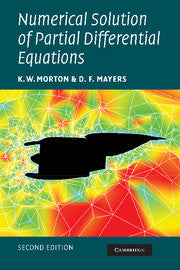Numerical Solution of Partial Differential Equations : An Introduction by Morton, K. W.

• R 489.00
Unit price per
Tax included.

ISBN: 9780521607933

Cambridge University Press | 07 February 2013

Paperback | 294 pages

This is the 2005 second edition of a highly successful and well-respected textbook on the numerical techniques used to solve partial differential equations arising from mathematical models in science, engineering and other fields. The authors maintain an emphasis on finite difference methods for simple but representative examples of parabolic, hyperbolic and elliptic equations from the first edition. However this is augmented by new sections on finite volume methods, modified equation analysis, symplectic integration schemes, convection-diffusion problems, multigrid, and conjugate gradient methods; and several sections, including that on the energy method of analysis, have been extensively rewritten to reflect modern developments. Already an excellent choice for students and teachers in mathematics, engineering and computer science departments, the revised text includes more latest theoretical and industrial developments.